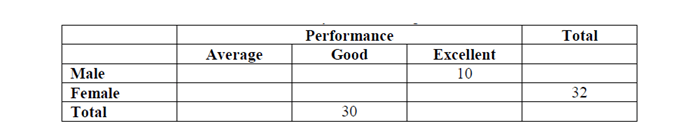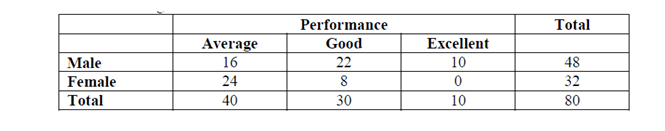DIRECTION : A professor keeps data on students tabulated by performance and sex of the student. The data is kept on a computer disk, but unfortunately some of it is lost because of a virus. Only the following could be recovered:Panic buttons were pressed but to no avail. An expert committee was formed, which decided that the following facts were self evident:
1.One third of the male students were average.
2.Half the students were either excellent or good.
3.40% of the students were females.

Question 1. How many students are both male and good?
(a) 10
(b) 16
(c) 22
(d) 48

Question 2. How many students were both female and excellent?
(a) 0
(b) 8
(c) 16
(d) 32

Question 3. What proportion of female students are good?
(a) 0
(b) 0.25
(c) 0.5
(d) 1.0

Question 4. Among average students, what is the ratio of male to female?
(a) 1 : 2
(b) 2 : 1
(c) 3 : 2
(d) 2 : 3

Question 5. What proportion of good students are male?
(a) 0
(b) 0.73
(c) 0.4
(d) 1.0

1.  From the table it is given that the number of females are 32 which is the 40% of the student which means the total number of students are 80 and the number of boys are 48 2. Now in the question we are given by that half the students were excellent or good Also it is given that One third of the male students were average. So the numbers of male average students are 16 3. (Number of average students) = (number of good students + number of excellent students) = 40. 4. Total good students = 30 5. Therefore the number of excellent students = 40 – 30 = 10. 6. Also in the question it is mentioned that 1/3rd of male students were average, Therefore total number of boy students that were good = (48 – 16 – 10) = 22.

Now from this information our graph will change to the following graphNumber of students who are both male and good = 22.

Number of students who are both female & excellent = 0.

Proportion of female students who are good = (8/32) = 0.25.